Test: Circles- Case Based Type Questions

# Test: Circles- Case Based Type Questions - EmSAT Achieve

Test Description

## 12 Questions MCQ Test Mathematics for EmSAT Achieve - Test: Circles- Case Based Type Questions

Test: Circles- Case Based Type Questions for EmSAT Achieve 2023 is part of Mathematics for EmSAT Achieve preparation. The Test: Circles- Case Based Type Questions questions and answers have been prepared according to the EmSAT Achieve exam syllabus.The Test: Circles- Case Based Type Questions MCQs are made for EmSAT Achieve 2023 Exam. Find important definitions, questions, notes, meanings, examples, exercises, MCQs and online tests for Test: Circles- Case Based Type Questions below.
Solutions of Test: Circles- Case Based Type Questions questions in English are available as part of our Mathematics for EmSAT Achieve for EmSAT Achieve & Test: Circles- Case Based Type Questions solutions in Hindi for Mathematics for EmSAT Achieve course. Download more important topics, notes, lectures and mock test series for EmSAT Achieve Exam by signing up for free. Attempt Test: Circles- Case Based Type Questions | 12 questions in 24 minutes | Mock test for EmSAT Achieve preparation | Free important questions MCQ to study Mathematics for EmSAT Achieve for EmSAT Achieve Exam | Download free PDF with solutions
 1 Crore+ students have signed up on EduRev. Have you?
Test: Circles- Case Based Type Questions - Question 1

### Rohan draws a circle of radius 10 cm with the help of compass and scale. He also draws two chords, AB and CD in such a way that AB and CD are 6 cm and 8 cm from the centre O. Now, he has some doubts that are given below. Help him out by answering these questions: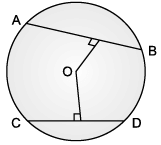Q. A quadrilateral is called cyclic if all the four vertices of it lie on a _________

Detailed Solution for Test: Circles- Case Based Type Questions - Question 1
A cyclic quadrilateral is a quadrilateral which has all its four vertices lying on a circle. It is also sometimes called inscribed quadrilateral.
Test: Circles- Case Based Type Questions - Question 2

### Rohan draws a circle of radius 10 cm with the help of compass and scale. He also draws two chords, AB and CD in such a way that AB and CD are 6 cm and 8 cm from the centre O. Now, he has some doubts that are given below. Help him out by answering these questions:Q. What is the length of CD?

Detailed Solution for Test: Circles- Case Based Type Questions - Question 2
Length of CD = 12 cm

h2 = p2 + b2

(10)2 = 82 + b2

b2 = 100 – 64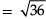b = 6 cm

CD = 6 + 6

= 12 cm

Test: Circles- Case Based Type Questions - Question 3

### Rohan draws a circle of radius 10 cm with the help of compass and scale. He also draws two chords, AB and CD in such a way that AB and CD are 6 cm and 8 cm from the centre O. Now, he has some doubts that are given below. Help him out by answering these questions:Q. What is the length of AB?

Detailed Solution for Test: Circles- Case Based Type Questions - Question 3
Length of AB = 16 cm

h2 = p2 + b2

102 = 62 + b2

100 = 36 + b2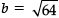= 8 cm

AB = 8 + 8

= 16 cm

Test: Circles- Case Based Type Questions - Question 4

Rohan draws a circle of radius 10 cm with the help of compass and scale. He also draws two chords, AB and CD in such a way that AB and CD are 6 cm and 8 cm from the centre O. Now, he has some doubts that are given below. Help him out by answering these questions:Q. A circle divides the plane, on which it lies, in _________ parts.

Detailed Solution for Test: Circles- Case Based Type Questions - Question 4
parts (inside, outside and on the circle)
Test: Circles- Case Based Type Questions - Question 5

Rohan draws a circle of radius 10 cm with the help of compass and scale. He also draws two chords, AB and CD in such a way that AB and CD are 6 cm and 8 cm from the centre O. Now, he has some doubts that are given below. Help him out by answering these questions:Q. Which statement is not true?

Detailed Solution for Test: Circles- Case Based Type Questions - Question 5
The sum of each pair of opposite angles of a cyclic quadrilateral is 90°.
Test: Circles- Case Based Type Questions - Question 6

A Ferris wheel (or a big wheel in the United Kingdom) is an amusement ride consisting of a rotating upright wheel with multiple passenger-carrying components (commonly referred to as passenger cars, cabins, tubs, capsules, gondolas, or pods) attached to the rim in such a way that as the wheel turns, they are kept upright, usually by gravity.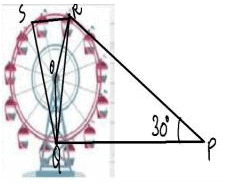After taking a ride in Ferris wheel, Aarti came out from the crowd and was observing her friends who were enjoying the ride . She was curious about the different angles and measures that the wheel will form. She forms the figure as given below.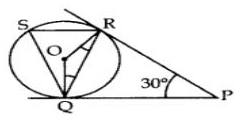Q. Find ∠ORP

Detailed Solution for Test: Circles- Case Based Type Questions - Question 6
PR = PO [∵ Tangents drawn from an external point are equal

⇒ ∠PRQ = ∠PQR [∵ Angles opposite equal sides are equal

In ∆PQR,

⇒ ∠PRQ + ∠RPQ + ∠POR = 180° [∆ Rule]

⇒ 30° + 2∠PQR = 180°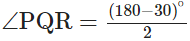= 75° ⇒ SR || QP and QR is a transversal

∵ ∠SRQ = ∠PQR [Alternate interior angle]

∴ ∠SRO = 75° [Tangent is I to the radius through the point of contact]

⇒ ∠ORP = 90°

∴ ∠ORP = ∠ORQ + ∠QRP

90° = ∠ORQ + 75°

∠ORQ = 90° – 75o = 150

Similarly, ∠RQO = 15°

In ∆QOR,

∠QOR + ∠QRO + ∠OQR = 180° [∆ Rule]

∴ ∠QOR + 15° + 15° = 180°

∠QOR = 180° – 30° = 150°

⇒ ∠QSR = 12∠QO

⇒ ∠QSR = 150/2 = 750 [Used ∠SRQ = 75° as solved above]

In ARSQ, ∠RSQ + ∠QRS + ∠RQS = 180° [∆ Rule]

∴ 75° + 75° + ∠RQS = 180°

∠RQS = 180° – 150o = 30°

Test: Circles- Case Based Type Questions - Question 7

A Ferris wheel (or a big wheel in the United Kingdom) is an amusement ride consisting of a rotating upright wheel with multiple passenger-carrying components (commonly referred to as passenger cars, cabins, tubs, capsules, gondolas, or pods) attached to the rim in such a way that as the wheel turns, they are kept upright, usually by gravity.After taking a ride in Ferris wheel, Aarti came out from the crowd and was observing her friends who were enjoying the ride . She was curious about the different angles and measures that the wheel will form. She forms the figure as given below.Q. Find ∠RQP

Detailed Solution for Test: Circles- Case Based Type Questions - Question 7
PR = PO [∵ Tangents drawn from an external point are equal

⇒ ∠PRQ = ∠PQR [∵ Angles opposite equal sides are equal

In ∆PQR,

⇒ ∠PRQ + ∠RPQ + ∠POR = 180° [∆ Rule]

⇒ 30° + 2∠PQR = 180°= 75° ⇒ SR || QP and QR is a transversal

∵ ∠SRQ = ∠PQR [Alternate interior angle]

∴ ∠SRO = 75° [Tangent is I to the radius through the point of contact]

⇒ ∠ORP = 90°

∴ ∠ORP = ∠ORQ + ∠QRP

90° = ∠ORQ + 75°

∠ORQ = 90° – 75o = 150

Similarly, ∠RQO = 15°

In ∆QOR,

∠QOR + ∠QRO + ∠OQR = 180° [∆ Rule]

∴ ∠QOR + 15° + 15° = 180°

∠QOR = 180° – 30° = 150°

⇒ ∠QSR = 12∠QO

⇒ ∠QSR = 150/2 = 750 [Used ∠SRQ = 75° as solved above]

In ARSQ, ∠RSQ + ∠QRS + ∠RQS = 180° [∆ Rule]

∴ 75° + 75° + ∠RQS = 180°

∠RQS = 180° – 150o = 30°

Test: Circles- Case Based Type Questions - Question 8

A Ferris wheel (or a big wheel in the United Kingdom) is an amusement ride consisting of a rotating upright wheel with multiple passenger-carrying components (commonly referred to as passenger cars, cabins, tubs, capsules, gondolas, or pods) attached to the rim in such a way that as the wheel turns, they are kept upright, usually by gravity.After taking a ride in Ferris wheel, Aarti came out from the crowd and was observing her friends who were enjoying the ride . She was curious about the different angles and measures that the wheel will form. She forms the figure as given below.Q. In the given figure find ∠ROQ

Detailed Solution for Test: Circles- Case Based Type Questions - Question 8
PR = PO [∵ Tangents drawn from an external point are equal

⇒ ∠PRQ = ∠PQR [∵ Angles opposite equal sides are equal

In ∆PQR,

⇒ ∠PRQ + ∠RPQ + ∠POR = 180° [∆ Rule]

⇒ 30° + 2∠PQR = 180°= 75° ⇒ SR || QP and QR is a transversal

∵ ∠SRQ = ∠PQR [Alternate interior angle]

∴ ∠SRO = 75° [Tangent is I to the radius through the point of contact]

⇒ ∠ORP = 90°

∴ ∠ORP = ∠ORQ + ∠QRP

90° = ∠ORQ + 75°

∠ORQ = 90° – 75o = 150

Similarly, ∠RQO = 15°

In ∆QOR,

∠QOR + ∠QRO + ∠OQR = 180° [∆ Rule]

∴ ∠QOR + 15° + 15° = 180°

∠QOR = 180° – 30° = 150°

⇒ ∠QSR = 12∠QO

⇒ ∠QSR = 150/2 = 750 [Used ∠SRQ = 75° as solved above]

In ARSQ, ∠RSQ + ∠QRS + ∠RQS = 180° [∆ Rule]

∴ 75° + 75° + ∠RQS = 180°

∠RQS = 180° – 150o = 30°

Test: Circles- Case Based Type Questions - Question 9

A Ferris wheel (or a big wheel in the United Kingdom) is an amusement ride consisting of a rotating upright wheel with multiple passenger-carrying components (commonly referred to as passenger cars, cabins, tubs, capsules, gondolas, or pods) attached to the rim in such a way that as the wheel turns, they are kept upright, usually by gravity.After taking a ride in Ferris wheel, Aarti came out from the crowd and was observing her friends who were enjoying the ride . She was curious about the different angles and measures that the wheel will form. She forms the figure as given below.Q. Find ∠RSQ

Detailed Solution for Test: Circles- Case Based Type Questions - Question 9
PR = PO [∵ Tangents drawn from an external point are equal

⇒ ∠PRQ = ∠PQR [∵ Angles opposite equal sides are equal

In ∆PQR,

⇒ ∠PRQ + ∠RPQ + ∠POR = 180° [∆ Rule]

⇒ 30° + 2∠PQR = 180°= 75° ⇒ SR || QP and QR is a transversal

∵ ∠SRQ = ∠PQR [Alternate interior angle]

∴ ∠SRO = 75° [Tangent is I to the radius through the point of contact]

⇒ ∠ORP = 90°

∴ ∠ORP = ∠ORQ + ∠QRP

90° = ∠ORQ + 75°

∠ORQ = 90° – 75o = 150

Similarly, ∠RQO = 15°

In ∆QOR,

∠QOR + ∠QRO + ∠OQR = 180° [∆ Rule]

∴ ∠QOR + 15° + 15° = 180°

∠QOR = 180° – 30° = 150°

⇒ ∠QSR = 12∠QO

⇒ ∠QSR = 150/2 = 750 [Used ∠SRQ = 75° as solved above]

In ARSQ, ∠RSQ + ∠QRS + ∠RQS = 180° [∆ Rule]

∴ 75° + 75° + ∠RQS = 180°

∠RQS = 180° – 150o = 30°

Test: Circles- Case Based Type Questions - Question 10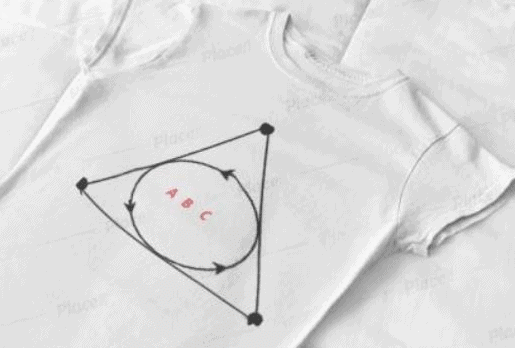Varun has been selected by his School to design logo for Sports Day T-shirts for students and staff . The logo design is as given in the figure and he is working on the fonts and different colours according to the theme. In given figure, a circle with centre O is inscribed in a ΔABC, such that it touches the sides AB, BC and CA at points D, E and F respectively. The lengths of sides AB, BC and CA are 12 cm, 8 cm and 10 cm respectively.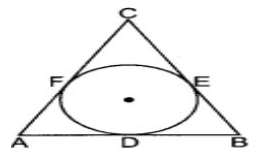Q. If radius of the circle is 4cm, Find the area of ∆OAB

Detailed Solution for Test: Circles- Case Based Type Questions - Question 10
Area ∆OAB = (1/2) * perpendicular height * Base = (1/2) * OD * AB = (1/2) * 4 * 12 = 24 cm²
Test: Circles- Case Based Type Questions - Question 11Varun has been selected by his School to design logo for Sports Day T-shirts for students and staff . The logo design is as given in the figure and he is working on the fonts and different colours according to the theme. In given figure, a circle with centre O is inscribed in a ΔABC, such that it touches the sides AB, BC and CA at points D, E and F respectively. The lengths of sides AB, BC and CA are 12 cm, 8 cm and 10 cm respectively.Q. Find the Length of BE

Detailed Solution for Test: Circles- Case Based Type Questions - Question 11
(x + y + z) - (x + z) = y => 15 - 10 = 5 cm = BE
Test: Circles- Case Based Type Questions - Question 12Varun has been selected by his School to design logo for Sports Day T-shirts for students and staff . The logo design is as given in the figure and he is working on the fonts and different colours according to the theme. In given figure, a circle with centre O is inscribed in a ΔABC, such that it touches the sides AB, BC and CA at points D, E and F respectively. The lengths of sides AB, BC and CA are 12 cm, 8 cm and 10 cm respectively.Q. Find area of ∆ABC

Detailed Solution for Test: Circles- Case Based Type Questions - Question 12
Area ∆ABC = Area ∆OAB + Area ∆OBC + Area ∆OCA

=> Area ∆ABC = [(1/2) * radius * AB] + [(1/2) * radius * BC] + [(1/2) * radius * CA]

=> Area ∆ABC = (1/2) * radius * (AB + BC + CA)

=> Area ∆ABC = (1/2) * 4 * 30

=> Area ∆ABC = 2 * 30

=> Area ∆ABC = 60 cm²

## Mathematics for EmSAT Achieve

151 videos|237 docs|254 tests
Information about Test: Circles- Case Based Type Questions Page
In this test you can find the Exam questions for Test: Circles- Case Based Type Questions solved & explained in the simplest way possible. Besides giving Questions and answers for Test: Circles- Case Based Type Questions, EduRev gives you an ample number of Online tests for practice

## Mathematics for EmSAT Achieve

151 videos|237 docs|254 tests